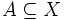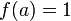# Tychonoff space

Jump to: navigation, search

## Definition

A topological space is termed a Tychonoff space if it satisfies the following equivalent conditions:

No. Shorthand A topological space is termed Tychonoff if ... A topological space$X$ is termed Tychonoff if ...
1 T1 and completely regular it is both a T1 space and a completely regular space points are closed in$X$, and given any point$x \in X$ and closed subset$A \subseteq X$ such that$x \notin A$, there exists a continuous map$f:X \to [0,1]$ such that$f(x) = 0$ and$f(a) = 1$ for all$a \in A$.
2 Hausdorff and completely regular it is both a Hausdorff space and a completely regular space it is Hausdorff, and given any point$x \in X$ and closed subset$A \subseteq X$ such that$x \notin A$, there exists a continuous map$f:X \to [0,1]$ such that$f(x) = 0$ and$f(a) = 1$ for all$a \in A$.
3 has a compactification there is a compact Hausdorff space having a dense subspace (with the subspace topology) homeomorphic to it. (note: T1 assumption redundant in this case) there is a compact Hausdorff space$C$ and a dense subspace$U$ of$X$ such that$X$ is homeomorphic to$U$.
4 contained in compact Hausdorff it is homeomorphic to a subspace (not necessarily dense) of a compact Hausdorff space (note: T1 assumption redundant in this case). there is a compact Hausdorff space$C$ and a subspace$U$ of$X$ such that$X$ is homeomoephic to$U$.
This article defines a property of topological spaces: a property that can be evaluated to true/false for any topological space|View a complete list of properties of topological spaces

In the T family (properties of topological spaces related to separation axioms), this is called: T3.5

## Relation with other properties

### Stronger properties

Property Meaning Proof of implication Proof of strictness (reverse implication failure) Intermediate notions
normal Hausdorff space Hausdorff and a normal space: disjoint closed subsets can be separated via disjoint open subsets |FULL LIST, MORE INFO
compact Hausdorff space Hausdorff and a compact space: every open cover has a finite subcover via compact Hausdorff implies normal Paracompact Hausdorff space|FULL LIST, MORE INFO
underlying space of T0 topological group |FULL LIST, MORE INFO
metrizable space underlying topological space of a metric space Paracompact Hausdorff space|FULL LIST, MORE INFO

### Weaker properties

Property Meaning Proof of implication Proof of strictness (reverse implication failure) Intermediate notions
completely regular space |FULL LIST, MORE INFO
regular Hausdorff space |FULL LIST, MORE INFO
regular space Regular Hausdorff space|FULL LIST, MORE INFO
Hausdorff space Functionally Hausdorff space, Regular Hausdorff space, Urysohn space|FULL LIST, MORE INFO